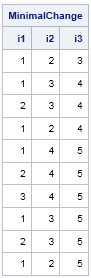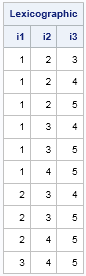In a previous blog post, I described how to generate combinations in SAS by using the ALLCOMB function in SAS/IML software. The ALLCOMB function in Base SAS is the equivalent function for DATA step programmers.

Recall that a combination is a unique arrangement of k elements chosen from a set of n elements. As such, the combination can always be written in a standard order where the elements increase from left to right and no element is larger than n. The output for the ALLCOMB function is shown to the left for n = 5 and k = 3.

As you scan down the rows, you might ask yourself, "In what order are these combinations generated?" The rows are not in the order that I would generate with pencil and paper. I would start with combination {1 2 3}, then proceed to increment the rightmost digit to obtain {1 2 4} and {1 2 5}. From there, I'd move over to the second-to-last column, increment that digit, reset the last column, and generate {1 3 4} and {1 3 5}. I would iteratively continue that process until all combinations were generated.

The pencil-and-paper method generates all of the combinations that begin with item 1, then generates the combinations that begin with item 2, and ends with the combination {3 4 5}. A list of combinations in "dictionary order," is called the lexicographic ordering. Clearly, the ALLCOMB function does not generate combinations in lexicographic order. Instead, the function generates the combinations in "minimal change order." Each combination is formed from the previous combination by removing one item and inserting another. Consequently, each row has k – 1 items in common with the preceding row.

SAS also provides a way to generate the combinations in lexicographic order. The following DATA step shows how to use the COMB function and LEXCOMBI function to generate the lexicographic ordering:

```%let n=5; /* total number of items */ %let k=3; /* number of items per row */ /* generate combinations of n items taken k at a time */ data Comb(keep=c1-c&k); array c[&k] (&k.*0); /* initialize array to 0 */ ncomb = comb(&n, &k); /* number of combinations */ do j = 1 to ncomb; rc = lexcombi(&n, &k, of c[*]); output; end; run;```The output is shown in the table on the right. Although it is not included in the output, the LEXCOMBI function returns the index of the column that was changed, which can be useful information for some applications.

One of the great things about SAS software is that there are multiple ways to accomplish most tasks. In addition to the preceding DATA step, you could also generate combinations in lexicographic order by doing any of the following:

### Programming the lexicographic combination algorithm

Most SAS programmers enjoy calling pre-written functions or procedures in SAS. However, SAS software provides the DATA step and the SAS/IML language for those occasions when you want to program an algorithm yourself.

This fact is especially pertinent for students and adult learners. With the launch of the free SAS University Edition, the next generation of SAS programmers has free access to the DATA step and the SAS/IML language. With a working knowledge of SAS programming, you can prepare data for analysis and implement new analyses that are not built into SAS.

The algorithm to "generate all combinations of n items taken k at a time," is a great programming exercise. Programming the algorithm without calling the LEXCOMBI function enables a programmer to gain experience processing DATA step arrays with k elements. It requires some subtle programming techniques, such as looping forward and backwards through indices of an array.

Would you like to try your hand at writing such a DATA step program? If so, stop reading now. Spoiler ahead!

The following DATA step was sent to me by a senior executive at SAS who still enjoys finding time to program. I found it easy to mentally trace through the program's logic for n = 5 and k = 3 to see how the program works. It keeps track of the number of combinations that it generates and prints the value of "n choose k" when the program terminates.

```/* Jim Goodnight's program to generate combinations (in lexicographic order) of n items taken k at a time */ data Comb(keep=c1-c&k); array c[&k]; /* array to hold combinations */ n=&n; k=&k; do i=1 to k; c[i]=i; end; /* initialize array to 1--k */ output;   count=1; /* enumerate combinations */ do while(count); /* find next combination */ if c[k]<n then c[k]+1; /* increment value in last column */ else do; n1=n; found=0; /* search earlier in array */ do i=k-1 to 1 by -1 while(found=0); n1=n1-1; if c[i]<n1 then found=i; /* found column to increment */ end; if found=0 then goto term; /* none found ... finished */ c[found]+1; /* increment value in found column */ do j=found+1 to k; c[j]=c[j-1]+1; /* reset values of subsequent cols */ end; end; output; count+1; end; term: put count=; /* at end, print count = comb(n,k) */ run;```

Can you modify the program to generate permutations in lexicographic order (see the LEXPERM function)? How would you vectorize this algorithm for the SAS/IML language? Do SAS programmers at your company implement algorithms like this, or do they mostly use the DATA step to extract, merge, transform, and prepare data for analysis? Leave a comment.

ShareDistinguished Researcher in Computational Statistics

Rick Wicklin, PhD, is a distinguished researcher in computational statistics at SAS and is a principal developer of SAS/IML software. His areas of expertise include computational statistics, simulation, statistical graphics, and modern methods in statistical data analysis. Rick is author of the books Statistical Programming with SAS/IML Software and Simulating Data with SAS.

1.Ian Wakeling on

Rick, you may well be better off with a solution that uses looping, but perhaps it could be vectorized in IML like this:
.
start comb(n,k);
fmt = cats('binary' , char(n,2,0) , '.');
y = num ( cshape( putn(0:2##n, fmt), 2##n, n, 1) );
y = y[ loc( y[ , +] = k), ];
y = 1 + shape( mod( loc(y) - 1, n), nrow(y) );
return(y);
finish;

print (comb(5,3));
.
Which works fine for small problems returning the combinations in reverse order. But as the module defines matrices with 2^n rows, unfortunately n = 22 is the maximum possible.

On my machine comb(23,3) gives an out of memory error message, and then declares that a negative number of extra bytes are required!